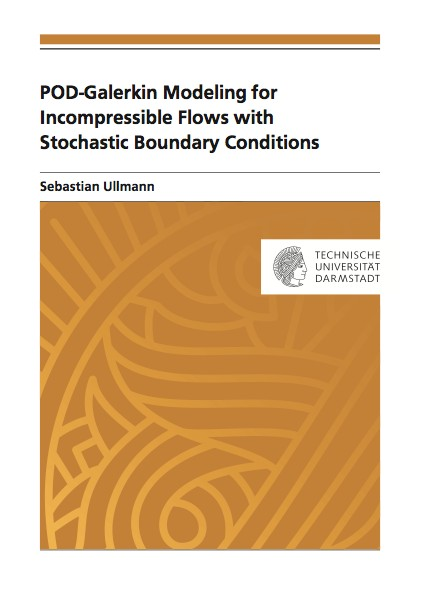Datenbestand vom 30. September 2022aktualisiert am 30. September 2022

# ISBN 978384391568772,00 € inkl. MwSt, zzgl. Versand

978-3-8439-1568-7, Reihe Mathematik

Sebastian Ullmann
POD-Galerkin Modeling for Incompressible Flows with Stochastic Boundary Conditions

181 Seiten, Dissertation Technische Universität Darmstadt (2014), Softcover, A5

## Zusammenfassung / Abstract

In the context of the numerical solution of parametrized partial differential equations, a proper orthogonal decomposition (POD) provides a basis of a subspace of the solution space. The method relies on a singular value decomposition of a snapshot matrix, which contains the numerical solutions at predefined parameter values. Often a sufficiently accurate representation of the solution can be given by a linear combination of a small number of POD basis functions. In this case, using POD basis functions as test and trial functions in a Galerkin projection leads to POD-Galerkin reduced-order models. Such models are derived and tested in this thesis for flow problems governed by the incompressible Navier-Stokes equations with stochastic Dirichlet boundary conditions.

In the first part of the thesis, POD-Galerkin reduced-order models are developed for unsteady deterministic problems of increasing complexity: heat conduction, isothermal flow, and thermoconvective flow. Here, time acts as a parameter, so that the snapshot matrix consists of discrete solutions at different times. Special attention is paid to the reduced-order computation of the pressure field, which is realized by projecting a discrete pressure Poisson equation onto a pressure POD basis. It is demonstrated that the reduced-order solutions of the considered problems converge toward the underlying snapshots when the dimension of the POD basis is increased.

The second part of the thesis is devoted to a steady thermally driven flow problem with a temperature Dirichlet boundary condition given by a spatially correlated random field. In order to compute statistical quantities of interest, the stochastic problem is split into separate deterministic sub-problems by means of a Karhunen-Loeve parametrization of the boundary data and subsequent stochastic collocation on a sparse grid. The sub-problems are solved with suitable POD-Galerkin models. Different methods to handle the parametrized Dirichlet conditions are introduced and compared. The use of POD-Galerkin reduced-order models leads to a significant speed-up of the overall computational process compared to a standard finite element model.